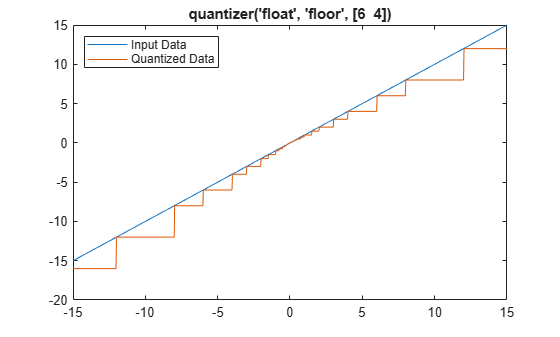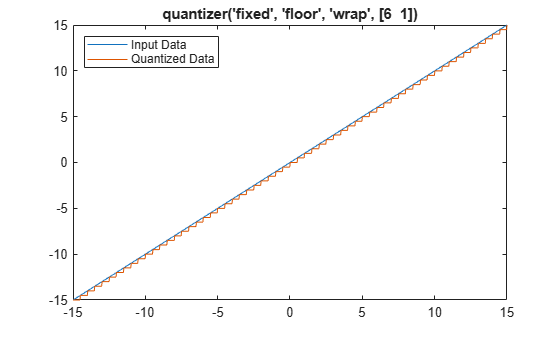# quantizer

Create `quantizer` object

## Description

The `quantizer` object describes data type properties to use for quantization. After you create a `quantizer` object, use `quantize` to quantize double-precision data. You can use the `quantizer` object to simulate custom floating-point data types with arbitrary word length and exponent length.

## Creation

### Syntax

``q = quantizer``
``q = quantizer(Name,Value)``
``q = quantizer(Value1,Value2)``
``q = quantizer(s)``
``q = quantizer(pn,pv)``

### Description

example

````q = quantizer` creates a `quantizer` object with properties set to their default values. To use this object to quantize values, use `quantize`.```

example

````q = quantizer(Name,Value)` sets named properties using name-value arguments. You can specify multiple name-value arguments. Enclose each property name in single quotes.```

example

````q = quantizer(Value1,Value2)` sets properties using property values. Property values are unique; you can set the property names by specifying just the property values in the command. When two values conflict, `quantizer` sets the last property value in the list.```

example

````q = quantizer(s)` sets properties named in each field name with the values contained in the structure `s`.```

example

````q = quantizer(pn,pv)` sets the named properties specified in the cell array of character vectors `pn` to the corresponding values in the cell array `pv`.You can use a combination of name-value string arguments, structures, and name-value cell array arguments to set property values when creating a `quantizer` object.```

## Properties

expand all

Data type mode used in quantization, specified as one of these values:

• `'fixed'` — Signed fixed-point mode.

• `'ufixed'` — Unsigned fixed-point mode.

• `'float'` — Custom-precision floating-point mode.

• `'single'` — Single-precision mode. This mode overrides all other property settings.

• `'double'` — Double-precision mode. This mode overrides all other property settings.

Data Types: `char` | `struct` | `cell`

Rounding method to use, specified as one of these values:

• `'ceil'` — Round up to the next allowable quantized value.

• `'convergent'` — Round to the nearest allowable quantized value. Numbers that are exactly halfway between the two nearest allowable quantized values are rounded up only if the least significant bit after rounding would be set to 0.

• `'fix'` — Round negative numbers up and positive numbers down to the next allowable quantized value.

• `'floor'` — Round down to the next allowable quantized value.

• `'nearest'` — Round to the nearest allowable quantized value. Numbers that are halfway between the two nearest allowable quantized values are rounded up.

• `'round'` — Round to the nearest allowable quantized value. Numbers that are halfway between the two nearest allowable quantized values are rounded up in absolute value.

Data Types: `char` | `struct` | `cell`

Action to take on overflow, specified as one of these values:

• `'saturate'` — Overflows saturate.

When the values of data to be quantized lie outside the range of the largest and smallest representable numbers as specified by the data format properties, these values are quantized to the value of either the largest or smallest representable value, depending on which is closest.

• `'wrap'` — Overflows wrap to the range of representable values.

When the values of data to be quantized lie outside the range of the largest and smallest representable numbers as specified by the data format properties, these values are wrapped back into that range using modular arithmetic relative to the smallest representable number.

This property only applies to fixed-point data type modes. This property becomes a read-only property when you set the `DataMode` property to `float`, `double`, or `single`.

Note

Floating-point numbers that extend beyond the dynamic range overflow to `±Inf`.

Data Types: `char` | `struct` | `cell`

Data format of `quantizer` object. The interpretation of this property value depends on the value of the `DataMode` property.

`DataMode` Property ValueInterpreting the Format Property Values
`fixed` or `ufixed`

`[wordlength fractionlength]`

Specify the `Format` property value as a two-element row vector, where the first element is the number of bits for the `quantizer` object word length and the second element is the number of bits for the `quantizer` object fraction length.

The word length can range from 2 to the limits of memory on your PC. The fraction length can range from 0 to one less than the word length.

`float`

`[wordlength exponenetlength]`

Specify the `Format` property value as a two-element row vector, where the first element is the number of bits for the `quantizer` object word length and the second element is the number of bits for the `quantizer` object exponent length.

The word length can range from 2 to the limits of memory on your PC. The fraction length can range from 0 to 11.

`double`

`[64 11]`

The read-only `Format` property value automatically specifies the word length and exponent length.

`single`

`[32 8]`

The read-only `Format` property value automatically specifies the word length and exponent length.

Data Types: `single` | `double` | `int8` | `int16` | `int32` | `int64` | `uint8` | `uint16` | `uint32` | `uint64`

### Read-Only `quantizer` Object States

Read-only `quantizer` object states are updated when `quantize` is called. To reset these states, use `reset`.

Maximum value before quantization during a call to `quantize(q,…)` for `quantizer` object `q`. This value is the maximum value recorded over successive calls to `quantize`.

Example: `max(q)`

Example: `q.max`

Minimum value before quantization during a call to `quantize(q,…)` for `quantizer` object `q`. This value is the minimum value recorded over successive calls to `quantize`.

Example: `min(q)`

Example: `q.min`

Number of overflows during a call to `quantize(q,…)` for `quantizer` object `q`. This value accumulates over successive calls to `quantize`. An overflow is defined as a value that when quantized is outside the range of `q`.

Example: `noverflows(q)`

Example: `q.noverflows`

Number of underflows during a call to `quantize(q,…)` for `quantizer` object `q`. This value accumulates over successive calls to `quantize`. An underflow is defined as a number that is nonzero before it is quantized and zero after it is quantized.

Example: `nunderflows(q)`

Example: `q.nunderflows`

Number of quantization operations during a call to `quantize(q,…)` for `quantizer` object `q`. This value accumulates over successive calls to `quantize`.

Example: `noperations(q)`

Example: `q.noperations`

## Object Functions

 `quantize` Quantize numeric data using `quantizer` object `unitquantize` Quantize numeric data using `quantizer` object except numbers within `eps` of `+1` `wordlength` Word length of `quantizer` object

## Examples

collapse all

Create a `quantizer` object with default property values.

`q = quantizer`
```q = DataMode = fixed RoundMode = floor OverflowMode = saturate Format = [16 15] ```

To copy a `quantizer` object, use assignment.

```q = quantizer; r = q; isequal(q,r)```
```ans = logical 1 ```

Use property name-value arguments to set `quantizer` object properties.

```q = quantizer('Mode','fixed','RoundMode','ceil',... 'OverflowMode','saturate','Format',[5 4])```
```q = DataMode = fixed RoundMode = ceil OverflowMode = saturate Format = [5 4] ```

Set `quantizer` object properties by listing property values only in the command.

`q = quantizer('fixed','ceil','saturate',[5 4])`
```q = DataMode = fixed RoundMode = ceil OverflowMode = saturate Format = [5 4] ```

Use a structure to set `quantizer` object properties.

```struct.DataMode = 'fixed'; struct.RoundMode = 'ceil'; struct.OverflowMode = 'saturate'; struct.Format = [5 4]; q = quantizer(struct)```
```q = DataMode = fixed RoundMode = ceil OverflowMode = saturate Format = [5 4] ```

Use property name and property value cell arrays to set `quantizer` object properties.

```pn = {'Mode','RoundMode','Overflowmode','Format'}; pv = {'fixed','ceil','saturate',[5 4]}; q = quantizer(pn,pv)```
```q = DataMode = fixed RoundMode = ceil OverflowMode = saturate Format = [5 4] ```

Use `quantize` to quantize data, see how quantization affects `quantizer` object states, and reset `quantizer` object states to their default values using `reset`.

Construct an example data set and create a `quantizer` object to specify the quantization parameters to use when you quantize the data set.

```format long g rng(0,'twister'); x = rng(100); q = quantizer([16,14])```
```q = DataMode = fixed RoundMode = floor OverflowMode = saturate Format = [16 14]```

Retrieve the values of `max` and `noverflows`.

```q.max q.noverflows```
```ans = -1.79769313486232e+308 ans = 0```

Note that `max` is equal to `-realmax`, which indicates that the quantizer `q` is in a reset state.

Use the `quantize` function to quantize the data set according to the specifications of the `quantizer` object.

`y = quantize(q,x);`
`Warning: 625 overflow(s) occurred in the fi quantize operation. `

Check the values of `max` and `noverflows`.

```q.max q.noverflows```
```ans = 1.99993896484375 ans = 625```

Note that the maximum logged value was taken after quantization, that is, `q.max == max(y)`.

Reset and check the `quantizer` states.

```reset(q) q.maxlog q.noverflows```
```ans = -1.79769313486232e+308 ans = 0```

This example shows how to quantize data using the properties specified by the `quantizer` object.

First, create some data to quantize.

`x = linspace(-15,15,1000);`

Quantize to Custom-Precision Floating-Point

Create a `quantizer` object specifying a custom-precision floating-point data mode with a word length of 6 bits and an exponent length of 4 bits.

`q = quantizer('DataMode','float','Format',[6 4])`
```q = DataMode = float RoundMode = floor Format = [6 4] ```

The `RoundMode` property uses the default setting of `'Floor'.`

Use the `quantize` function to quantize the data in `x` using the properties specified by the `quantizer` object.

`y = quantize(q,x);`

Plot `y` against `x` to visualize the effect of the specified quantization properties on this data.

```plot(x,x,x,y); title(tostring(q)); legend('Input Data','Quantized Data','Location','northwest');```You can use read-only properties of the `quantizer `object to access more information.

`q.noverflows`
```ans = 0 ```
`q.nunderflows`
```ans = 0 ```

In this example, there were 0 overflows and 0 underflows that occurred in the quantization operation.

Quantize to Fixed-Point

Create a `quantizer` object specifying a signed fixed-point data mode with a word length of 6 bits, a fraction length of 1 bit, and wrap on overflow.

`q = quantizer([6 1],'wrap')`
```q = DataMode = fixed RoundMode = floor OverflowMode = wrap Format = [6 1] ```

`quantizer` uses the default `DataMode` property, `'fixed'`, and the default `RoundMode` property, `'Floor'`.

Use the `quantize` function to quantize the data in `x` using the properties specified by the `quantizer` object.

`y = quantize(q,x);`

Plot `y` against `x` to visualize the effect of the specified quantization properties on this data.

```plot(x,x,x,y); title(tostring(q)); legend('Input Data','Quantized Data','Location','northwest');```You can use read-only properties of the `quantizer `object to access more information.

`q.noverflows`
```ans = 0 ```
`q.nunderflows`
```ans = 17 ```

In this example, there were 0 overflows and 17 underflows that occurred in the quantization operation.

## Version History

Introduced before R2006a